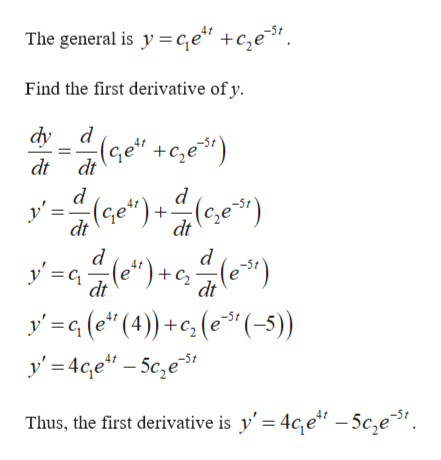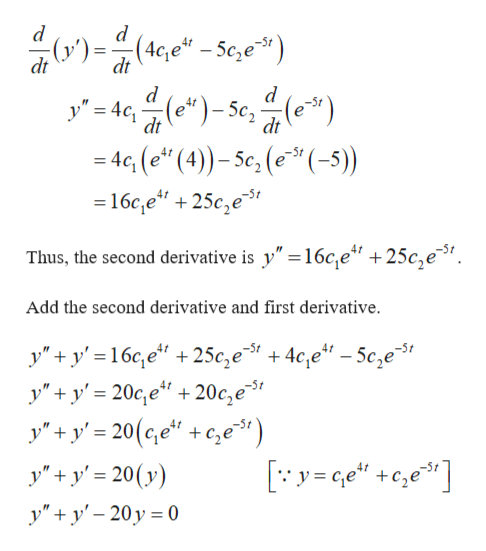# Find the differential equation whose general solution isy=c1e4t+c2e-5t

Question
2 views

Find the differential equation whose general solution is

y=c1e4t+c2e-5t

check_circle

Step 1

Find the differential equation of given solution.help_outlineImage TranscriptioncloseThe general is y ge" +c,e3, Find the first derivative ofy dy d :(Ge" +c,e) dt dt (Ge")- y' = dt dt y' ) y g (e (4)c (e3" (-5)) y'4ce-Sc,e* (e") dt -5t dt -5t Thus, the first derivative is y'= 4c,e" -5c,e31 fullscreen
Step 2

Find the second derivative of and use both deri...help_outlineImage Transcriptionclosed (v)= (4c,e - 5c,e*) dt dt y'=4c e")-5c, 4(e*) 4c(e" (4)-5c, ((-5) =16c,e" + 25c,e dt dt Thus, the second derivative is y" =16c,e" +25c,e. Add the second derivative and first derivative y" + y'=16c,e" + 25c,e* + 4c,e" - 5e,e"31 | y"y'20c,e 20c,e y' + y'= 20(ce" +c,e) y"y'20(y) y"y'20y 0 fullscreen

### Want to see the full answer?

See Solution

#### Want to see this answer and more?

Solutions are written by subject experts who are available 24/7. Questions are typically answered within 1 hour.*

See Solution
*Response times may vary by subject and question.
Tagged in

### Math